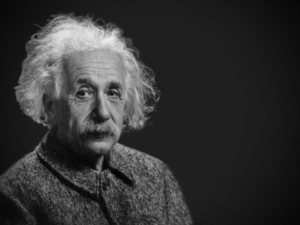# Theory of RelativityTheory of Relativity: Time and space are interrelated, space-time. Space and time are relative and not fixed.

Albert Einstein’s general theory of relativity is one of the towering achievements of 20th-century physics. Published in 1916, it explains that what we perceive as the force of gravity in fact arises from the curvature of space and time. Einstein proposed that objects such as the sun and the Earth change this geometry.(Feel Good Now p. 91)

All motion must be defined relative to a frame of reference and that space and time are relative, rather than absolute concepts: it consists of two principal parts.

E=mc2 explains nuclear fusion, how matter can be destroyed and converted to energy and energy can be converted back to mass. E = mc2.  E represents units of energy, m represents units of mass, and c2 is the speed of light squared, or multiplied by itself.

In Einstein’s theory of relativity, time dilation describes a difference of elapsed timebetween two events, as measured by observers that are either moving relative to each other, or differently, depending on their proximity to a gravitational mass.

‘Til the next time.-Rosie